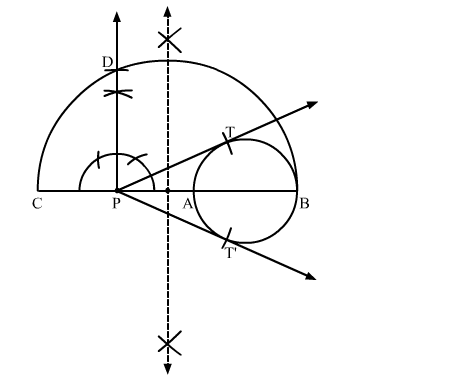# Draw a circle with the help of a bangle.`
Question:

Draw a circle with the help of a bangle. Take any point P outside the circle. Construct the pair of tangents from the point P to the circle.

Solution:

Steps of Construction

Step 1. Draw a circle with the help of a bangle.

Step 2. Mark a point P outside the circle.

Step 3. Through P, draw a secant PAB to intersect the circle at A and B.

Step 4. Produce AP to C such that PA = PC.

Step 5. Draw a semicircle with CB as diameter.

Step 6. Draw PD ⊥ BC, intersecting the semicircle at D.

Step 7. With P as centre and PD as radius, draw arcs to intersect the circle at T and T'.

Step 8. Join PT and PT'.Here, PT and PT' are the required pair of tangents.